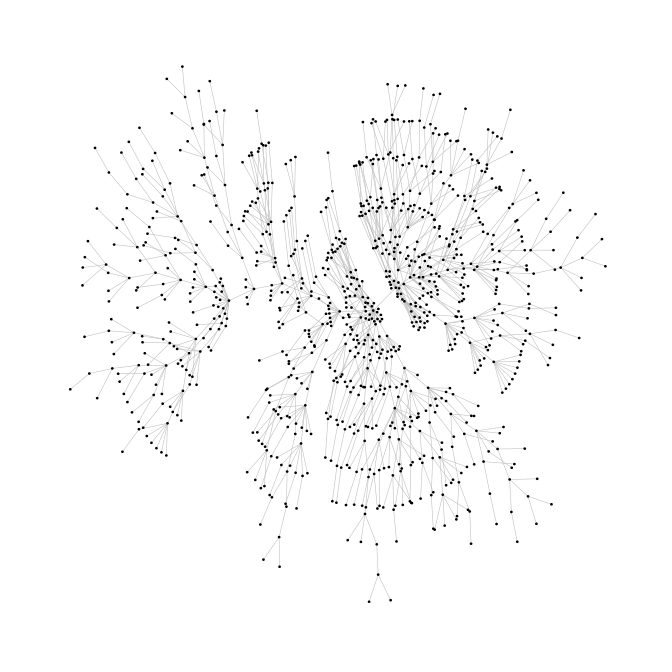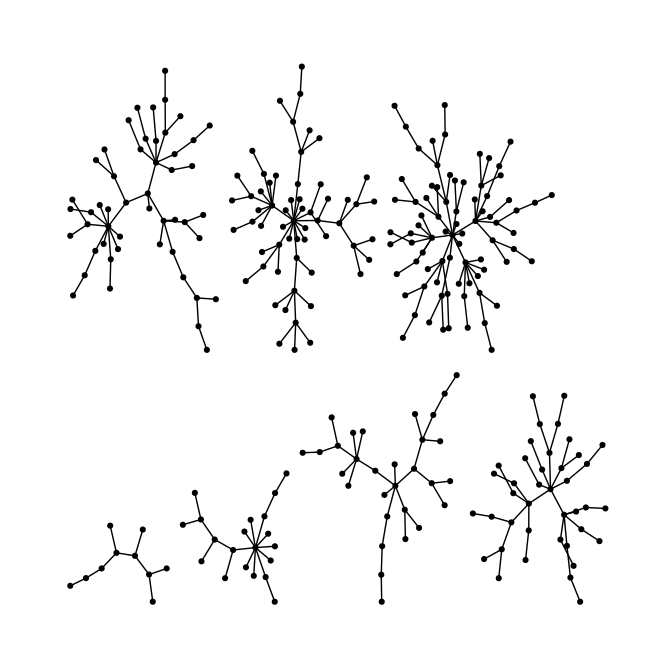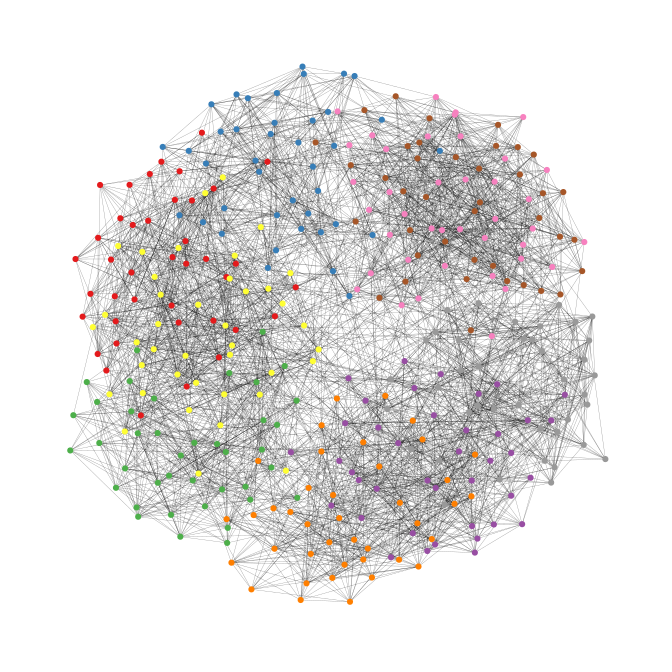# graphlayouts

This package implements some graph layout algorithms that are not available in `igraph` or other packages. This vignette only introduces the basic functionality of the package and the most important layout functions. A more in depth tutorial on network visualization in R using `graphlayouts` which introduces its full functionality can be found online.

``````library(igraph)
library(ggraph)
library(graphlayouts)``````

## Implemented layout algorithms

The package implements the following algorithms:

• Stress majorization (Paper)
• sparse stress (Paper)
• pivot MDS (Paper)
• dynamic layout for longitudinal data (Paper)
• a simple multilevel layout
• a layout algorithm using UMAP
• group based centrality and focus layouts which keeps groups of nodes close in the same range on the concentric circle

## Stress layout for connected network

The “stress” layout is always a safe layout choice since it is deterministic and produces nice plots for almost any graph. It is recommended to use it as a default choice.

``````set.seed(666)
pa <- sample_pa(1000,1,1,directed = F)

ggraph(pa,layout = "stress")+
geom_node_point(color = "black",size = 0.3)+
theme_graph()
#> Warning: Using the `size` aesthetic in this geom was deprecated in ggplot2 3.4.0.
#> ℹ Please use `linewidth` in the `default_aes` field and elsewhere instead.
#> This warning is displayed once every 8 hours.
#> Call `lifecycle::last_lifecycle_warnings()` to see where this warning was
#> generated.``````## Stress layout for unconnected network

Stress majorization also works for networks with several components. It relies on a bin packing algorithm to efficiently put the components in a rectangle, rather than a circle.

``````set.seed(666)
g <- disjoint_union(
sample_pa(10,directed = F),
sample_pa(20,directed = F),
sample_pa(30,directed = F),
sample_pa(40,directed = F),
sample_pa(50,directed = F),
sample_pa(60,directed = F),
sample_pa(80,directed = F)
)

ggraph(g, layout = "stress",bbox = 40) +
geom_node_point() +
theme_graph()``````## Backbone layout

`layout_as_backbone()` is a layout algorithm that can help emphasize hidden group structures. To illustrate the performance of the algorithm, we create an artificial network with a subtle group structure using `sample_islands()` from `igraph`.

The network consists of 9 groups with 40 vertices each. The density within each group is 0.4 and there are 15 edges running between each pair of groups. Using the recommended approach using the stress layout yields the following result.

``````set.seed(665)
#create network with a group structure
g <- sample_islands(9,40,0.4,15)
g <- simplify(g)
V(g)\$grp <- as.character(rep(1:9,each=40))

ggraph(g,layout = "stress")+
geom_node_point(aes(col = grp))+
scale_color_brewer(palette = "Set1")+
theme_graph()+
theme(legend.position = "none")``````As one can see, the graph seems to be a proper hairball without any special structural features standing out. In this case, though, we know that there should be 9 groups of vertices that are internally more densely connected than externally. To uncover this group structure, we turn to the backbone layout.

The backbone layout helps to uncover potential group structures based on edge embeddedness and puts more emphasis on this structure in the layout.

To use the function, you need to install the package `oaqc`

``install.packages("oaqc")``
``````bb <- layout_as_backbone(g,keep=0.4)
E(g)\$col <- F
E(g)\$col[bb\$backbone] <- T

ggraph(g,layout = "manual",x = bb\$xy[,1],y = bb\$xy[,2])+